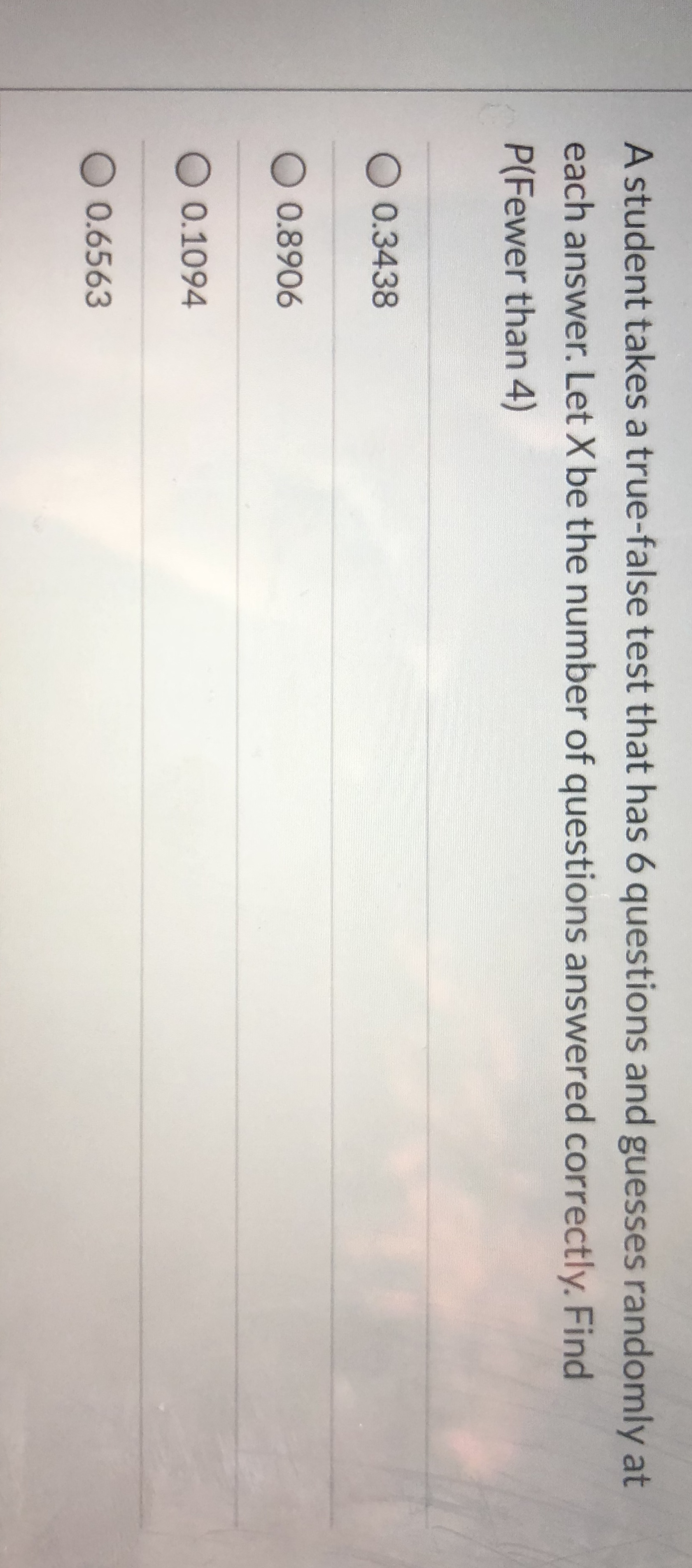# A student takes a true-false test that has 6 questions and guesses randomly at each answer. Let X be the number of questions answered correctly. Find P(Fewer than 4)

Questionhelp_outlineImage TranscriptioncloseA student takes a true-false test that has 6 questions and guesses randomly at each answer. Let X be the number of questions answered correctly. Find P(Fewer than 4) fullscreen

### Want to see this answer and more?

Experts are waiting 24/7 to provide step-by-step solutions in as fast as 30 minutes!*

*Response times may vary by subject and question complexity. Median response time is 34 minutes for paid subscribers and may be longer for promotional offers.
Tagged in
Math
Statistics

### Other